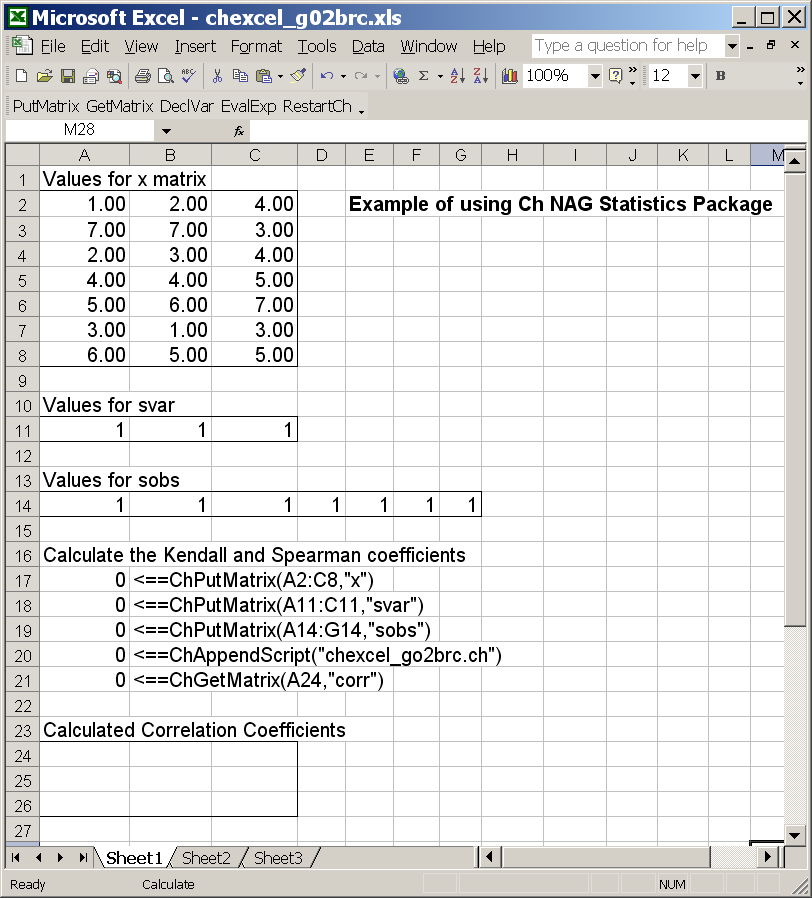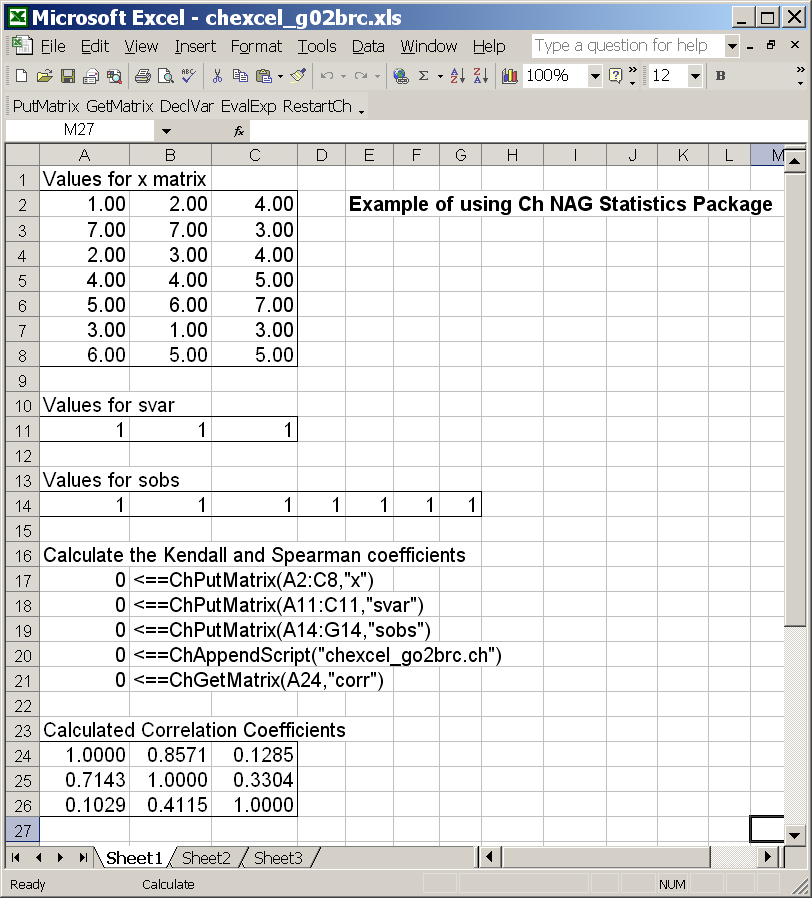SoftIntegration Demos for Ch and Toolkits
 Demos Ch Toolkit Demos Ch Mechanism Ch Control System Ch CGI Ch ODBC ChExcel C++ Library Demos Graphical Library Package Demos Ch NAG Statistics

Ch NAG Statisitics Demos.

This example illustrates how functions in Ch NAG Statisics Package are used in Excel through ChExcel.

Excel spreadsheet chexcel_g02brc.xls and Ch script chexcel_g02brc.ch for using function g02brc()() in Ch NAG Statistics described below are included in the distribution of Ch NAG Statisics Package. The values in certain cells of the Excel spreadsheet chexcel_g02brc.xls are passed to Ch script chexcel_g02brc.ch for processing and the result from the function is then placed in cells.

The program below shows Ch script chexcel_g02brc.ch.

/* program chexcel_g02brc.ch */ #include <nag.h> #include <stdio.h> #include <nag_stdlib.h> #include <nagg02.h> #include <array.h> /* variables x, svar, sobs are declared inside ChExcel chexcel_g02brc.xls array double x = { {1.0, 2.0, 4.0}, {7.0, 7.0, 3.0}, {2.0, 3.0, 4.0}, {4.0, 4.0, 5.0}, {5.0, 6.0, 7.0}, {3.0, 1.0, 3.0}, {6.0, 5.0, 5.0} }; double svar = {1, 1, 1}; double sobs = {1, 1, 1, 1, 1, 1, 1}; int n=7, m=3; */ array int dim = shape(x); int n = dim, m = dim; Integer i, j, tdx, tdc; array int svari[m]; array int sobsi[n]; double corr[m][m]; tdx = m; tdc = m; svari = svar; sobsi = sobs; /* Calculate the Kendall and Spearman coefficients */ g02brc(n, m, &x, tdx, &svari, &sobsi, &corr,tdc, NAGERR_DEFAULT); The program begins by including the header files #include <nag.h> #include <stdio.h> #include <nag_stdlib.h> #include <nagg02.h> #include <array.h> Variables x, svar, sobs are declared and initialized through inside ChExcel. These values are passed to function g02brc() to calculate the Kendall and Spearman correlation coefficients. The coefficients are kept in the variable corr.

The first figure below shows the spreadsheet chexcel_g02brc.xls. Type F2 from top to the bottom for 5 times by placing the cursor on "0" at the left below.

0 <==ChPutMatrix(A2:C8,"x") 0 <==ChPutMatrix(A11:C11,"svar") 0 <==ChPutMatrix(A14:G14,"sobs") 0 <==ChAppendScript("chexcel_go2brc.ch") 0 <==ChGetMatrix(A24,"corr")

The first three function calls declare and initialize variables x, svar, and sobs of array type. These variables are used inside program chexcel_go2brc.ch, which is executed by ChExcel function ChAppendScript("chexcel_go2brc.ch"). chexcel_g02brc.ch invoked from chexcel_g02brc.xls will call g02brc() to calculate correlation coefficients for an array input from Excel. The values in array corr declared inside chexcel_go2brc.ch are put in cells starting with A24 by calling ChExcel function ChGetMatrix(A24,"corr").

The Excel spreadsheet before calling ChExcel functions.The Excel spreadsheet after calling ChExcel functions.